# Multiplexed mass cytometry profiling of cellular states perturbed by small-molecule regulators

Single cell analysis is a powerful method that allows for the deconvolution of the effect of treatments on complex populations containing different cell types, that may or may not respond to specific treatments. Depending on the technology used, the analytes can be genes, transcripts, proteins or metabolites. Using mass cytometry, bodenmiller et al measured the level of 9 proteins and 14 post-translational modifications. After using signal intensity from the 9 proteins (so called phenotypic markers) to define 14 sub-populations, they monitored the effect of several treatments using the 14 post-translational modifications.

Modeling and visualization of these type of data is challenging: the large number of events measured combined to the complexity of each samples is making the modeling complex, while the high dimensionality of the data precludes the use of standard visualizations.

The goal of this package is to enable the development of new methods by providing a curated set of data for testing and benchmarking.

# Data acquisition and preparation

For details on data acquisition please refer to Bodenmiller et al Nat Biotech 2012. Briefly, after treatment cells where profiled using a CyTOF, dead cells and debris were excluded and live cells were assigned to 1 of the 14 sub-populations using signal intensity from 9 phenotypic markers.

Cells from samples samples corresponding to untreated cells, stimulated with BCR/FcR-XL, PMA/Ionomycin or vanadate or unstimulated, were extracted and data was transformed using the arcsinh function with a cofactor of 5.

Due to recent changes in Cytobank the original data is not available anymore, so this vignette relies on the bodenmiller package for access to data.

# Effects of stimulation on B and T cells

## Collecting single cell data

We begin by assembling a full dataset from the bodenmiller package, before filtering down to T cells:

data(refPhenoMat)
data(refFuncMat)
data(refAnnots)
ref.df <- data.frame(refAnnots,
refPhenoMat,
refFuncMat)

data(untreatedPhenoMat)
data(untreatedFuncMat)
data(untreatedAnnots)
untreated.df <- bind_rows(ref.df %>%
mutate(Treatment='unstimulated',
Source=as.character(Source),
Cells=as.character(Cells)),
data.frame(untreatedAnnots,
untreatedPhenoMat,
untreatedFuncMat) %>%
mutate(Treatment=as.character(Treatment),
Source=as.character(Source),
Cells=as.character(Cells))) %>%
mutate(Treatment=factor(Treatment),
Treatment=relevel(Treatment,'unstimulated'),
Cells=factor(Cells))

btcells.df <- untreated.df %>%
filter(Cells %in% c('cd8+','igm+')) %>%
mutate(Cells=droplevels(Cells)) %>%
group_by(Cells,Treatment) %>%
mutate(cellID=seq(length(Cells))) %>%
unite('cellID',one_of(c('Treatment','Cells','cellID')),sep = '_',remove = FALSE)

We end up with 19807 cells to analyse, broken down by stimulation condition as follows:

left_join(btcells.df %>%
count(Cells,Treatment),
untreated.df %>%
count(Treatment,name = 'Total'),
by=c('Treatment')) %>%
mutate(Fraction=round(100*n/Total,1))
## # A tibble: 8 x 5
## # Groups:   Cells, Treatment 
##   Cells Treatment         n Total Fraction
##   <fct> <fct>         <int> <int>    <dbl>
## 1 cd8+  unstimulated   5068 15792     32.1
## 2 cd8+  BCR/FcR-XL     5098 15252     33.4
## 3 cd8+  PMA/Ionomycin  5122 14576     35.1
## 4 cd8+  vanadate       1200  6316     19
## 5 igm+  unstimulated   1069 15792      6.8
## 6 igm+  BCR/FcR-XL      863 15252      5.7
## 7 igm+  PMA/Ionomycin   992 14576      6.8
## 8 igm+  vanadate        395  6316      6.3

The Vanadate condition seems to contain less cells, and the fraction of CD4+ T cells is roughly 25% of the unstimulated sample. There is also a drop in the fraction of CD8+ T cells.

To simplify processing we will sample 1000 cells from each condition, with replacement where appropriate:

btcells.df <- btcells.df %>%
group_by(Cells,Treatment) %>%
sample_n(500,replace = TRUE)

## CD8+ T and IgM+ B cells profile

We used fan plots to visualize the phenotypic and functional profiles of CD8+ T cells and IgM+ B cells. As expected there is no change in phenotype while stimulation is changing the functional profile of each population.

### Phenotypic

btcells.df %>%
gather('Channel','value',
one_of(colnames(refPhenoMat),colnames(refFuncMat))) %>%
filter(Channel %in% colnames(refPhenoMat)) %>%
ggplot(aes(x=Channel,y=value))+
geom_fan()+
facet_grid(Treatment~Cells)+
theme_light(base_size = 16)+
theme(axis.text.x = element_text(angle = 45, hjust = 1))
## Warning: guides(<scale> = FALSE) is deprecated. Please use guides(<scale> =
## "none") instead.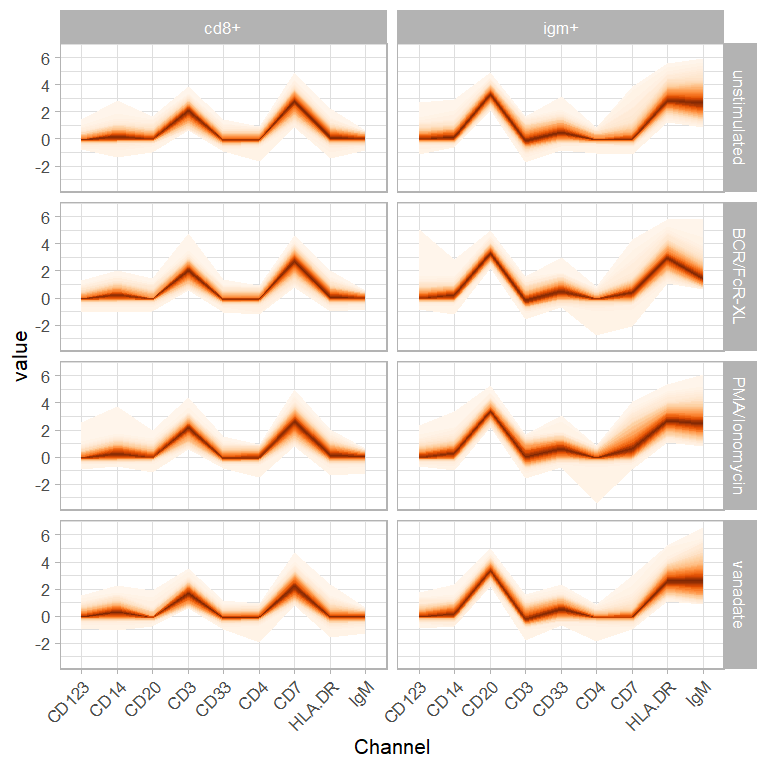### Functional

btcells.df %>%
gather('Channel','value',
one_of(colnames(refPhenoMat),colnames(refFuncMat))) %>%
filter(Channel %in% colnames(refFuncMat)) %>%
ggplot(aes(x=Channel,y=value))+
geom_fan()+
facet_grid(Treatment~Cells)+
theme_light(base_size = 16)+
theme(axis.text.x = element_text(angle = 45, hjust = 1))
## Warning: guides(<scale> = FALSE) is deprecated. Please use guides(<scale> =
## "none") instead.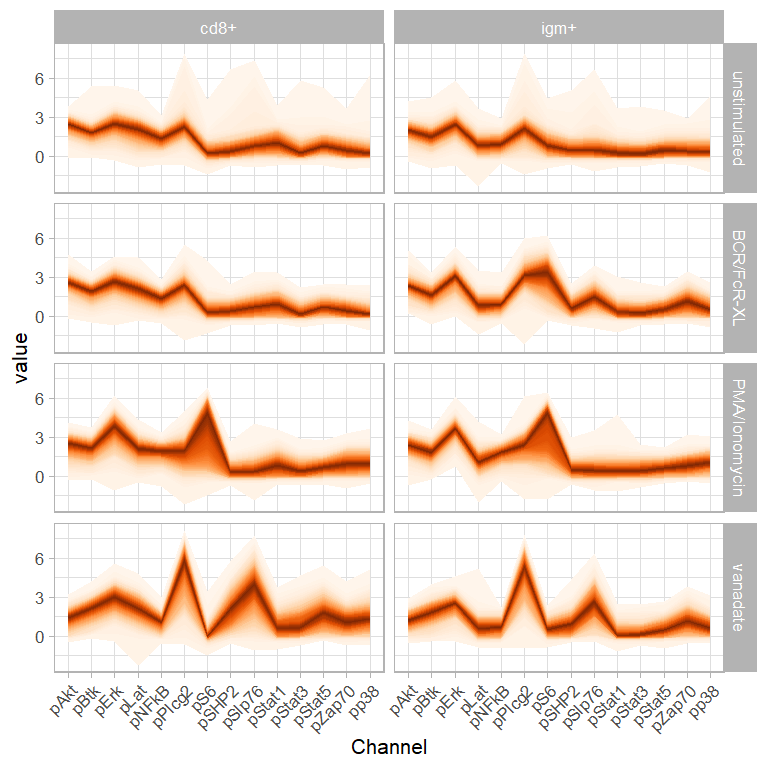## Selecting channels relevant to T cells

We select channels for analysis where 20% of cells from any treatment, any cell type, have a recorded intensity of at least 1 unit:

btcells.channels <- btcells.df %>%
gather('Channel','value',
one_of(colnames(refPhenoMat),colnames(refFuncMat))) %>%
group_by(Channel,Treatment,Cells) %>%
summarize(value=quantile(value,0.8)) %>%
group_by(Channel) %>%
summarise(value=max(value)) %>%
filter(value>1) %>%
.$Channel ## summarise() has grouped output by 'Channel', 'Treatment'. You can override using the .groups argument. The following 20 were selected out of 23: ksink <- lapply(btcells.channels,function(x) cat(' -',x,'\n')) • CD20 • CD3 • CD33 • CD7 • HLA.DR • IgM • pAkt • pBtk • pErk • pLat • pNFkB • pPlcg2 • pS6 • pSHP2 • pSlp76 • pStat1 • pStat3 • pStat5 • pZap70 • pp38 # Using Radviz to visualize the effects of stimulation We start by projecting cells using the classical Radviz algorithm: sim.mat <- cosine(as.matrix(btcells.df[,btcells.channels])) classic.S <- make.S(btcells.channels) classic.optim <- do.optimRadviz(classic.S,sim.mat) ## Selected optimization function: in.da ## Starting performance: -101.52 ## 0 Current performance: -104.1355 ## 1 Current performance: -104.9882 ## 2 Current performance: -105.6186 ## 3 Current performance: -106.0991 ## 4 Current performance: -107.0392 ## 5 Current performance: -107.0392 ## 6 Current performance: -107.0583 ## 7 Current performance: -107.5819 ## 8 Current performance: -107.8592 ## 9 Current performance: -107.8592 ## 10 Current performance: -108.3144 ## 11 Current performance: -108.4125 ## 12 Current performance: -108.4125 ## 13 Current performance: -108.4479 ## 14 Current performance: -108.4479 ## 15 Current performance: -108.4479 ## 16 Current performance: -108.4479 ## 17 Current performance: -108.4479 ## 18 Current performance: -108.4479 ## Execution stopped after 18 iterations: no better solution found in the last 5 iterations classic.S <- make.S(get.optim(classic.optim)) btcells.rv <- do.radviz(btcells.df,classic.S) Next we visualize the projection, coloring individual cells by their treatment of origin: plot(btcells.rv)+ geom_point(aes(color=Treatment))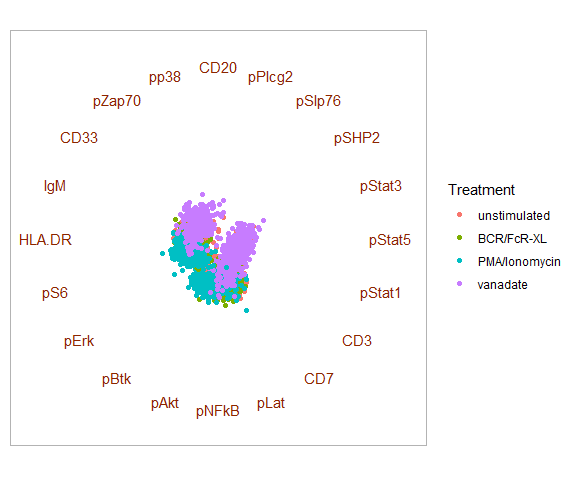Because of the spring paradigm used by Radviz the cells are concentrated to the center of the plot. Using the rescalePlot function we can zoom inside the circle : btcells.rv <- rescalePlot(btcells.rv) plot(btcells.rv)+ geom_point(aes(color=Treatment))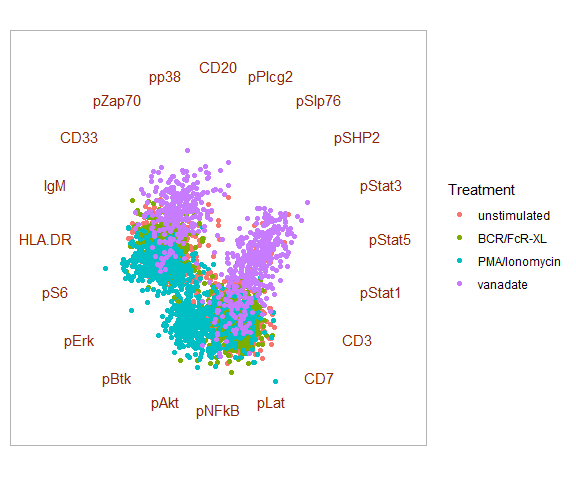We see a clear effect of Vanadate, and 2 separate groups of points. We confirm with the next plot that those points actually correspond to B and T cells: plot(btcells.rv)+ geom_point(aes(color=Cells))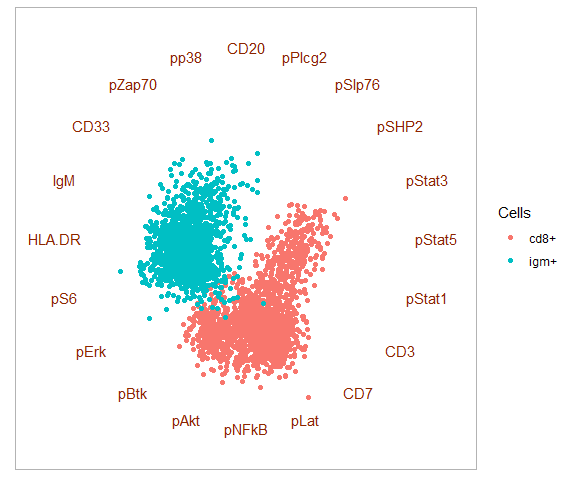One can facet the display by population to visualize the effects of stimulation: plot(btcells.rv)+ geom_point(aes(color=Treatment))+ facet_wrap(~Cells)+ theme_radviz(base_size = 16)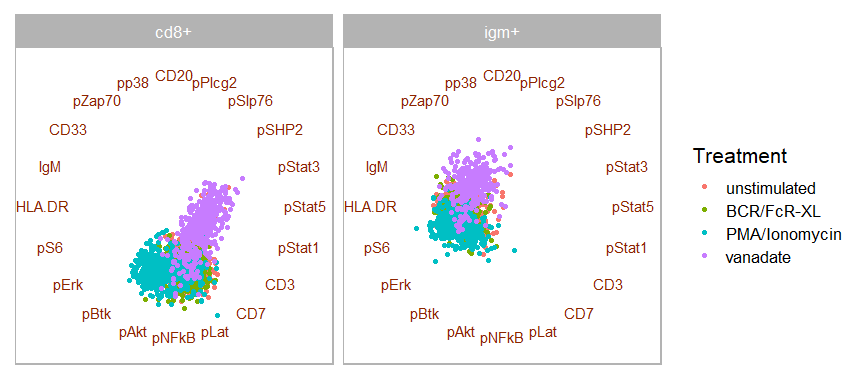We can now visualize the effects of Vanadate and Ionomycin stimulations compared to unstimulated, but the contributions of individual channels are unclear and the effects of BCR/FcR-XL on B cells are unclear. Moreover, the visualization depends on the optimized order of channels, which in turn depends on the relative amount of the different conditions. To address these challenges we implemented the Freeviz algorithm described in the next section. # Using Freeviz to visualize the effects of stimulation Freeviz will optimize the order of channels as well as their weights based on predefined classes in the data. In that example we use stimulation and cell type : btcells.df <- btcells.df %>% unite('Condition',c('Treatment','Cells'),sep='_',remove = FALSE) treat.S <- do.optimFreeviz(btcells.df[,btcells.channels], classes = btcells.df$Condition)
##  "# iters: 21"
btcells.fv <- do.radviz(btcells.df, treat.S)

The final projection is shown below, with cells colored by stimulation:

plot(btcells.fv)+
geom_point(aes(color=Treatment))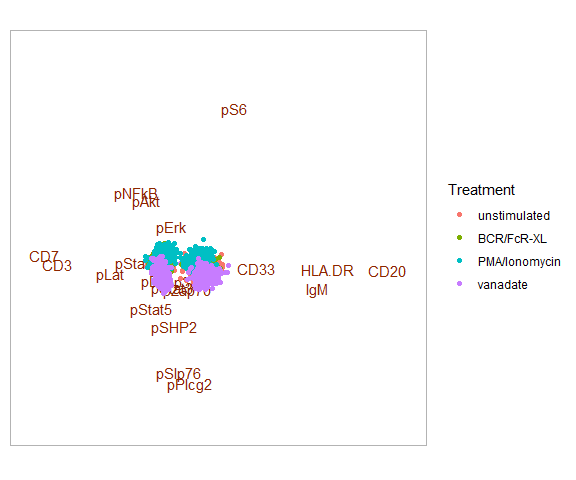Compared to Radviz, where the contribution of each channel is fixed through its position on the circle, in Freeviz the channels with the largest contribution to the difference between classes are the furthest away from the center of the projection. It is therefore easier to differentiate B cells from T cells, and to identify the channels that are affected the most by stimulation.

plot(btcells.fv)+
geom_point(aes(color=Treatment))+
facet_wrap(~Cells)+
theme_radviz(base_size = 16)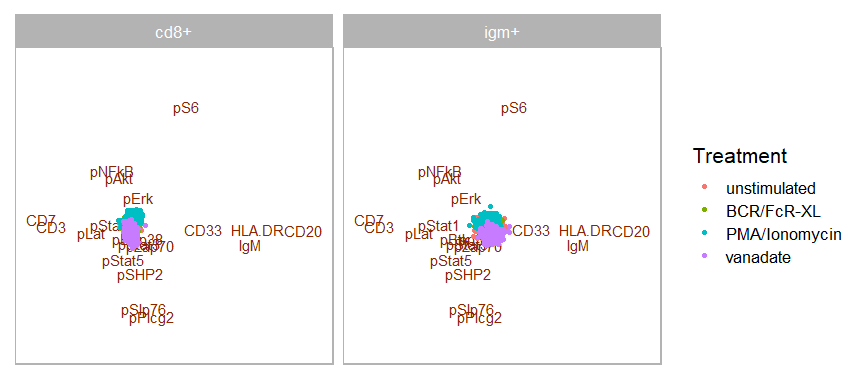Freeviz plots can also be rescaled : if after rescaling the points extend beyond a particular anchor, the exact contribution of this anchor to the projection is lost except for the direction. The function will issue a warning whenever this occurs.

btcells.fv <- rescalePlot(btcells.fv,fraction=0.5)
## Warning in rescalePlot(btcells.fv, fraction = 0.5): After rescaling the following anchors will be below the maximum range of points:
## pp38, pZap70, pStat3, pBtk, pErk, pStat1, pStat5, pSHP2, CD33, pLat, pAkt, pNFkB
plot(btcells.fv)+
geom_point(aes(color=Treatment))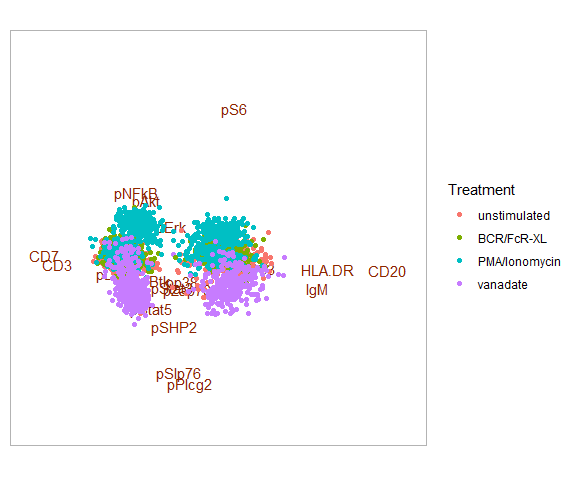After rescaling it is clear that a subset of B and T cells is not responding to the treatment:

contour(btcells.fv,
color='Treatment')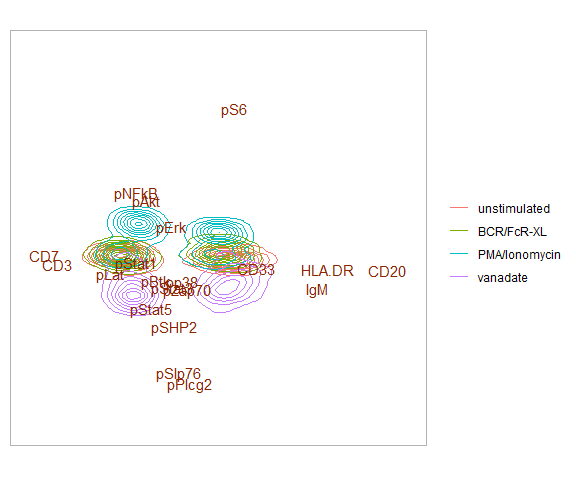In any case, it is possible to filter out the channels that have low influence on the projection:

plot(anchor.filter(btcells.fv,
lim=0.5))+
geom_point(aes(color=Treatment))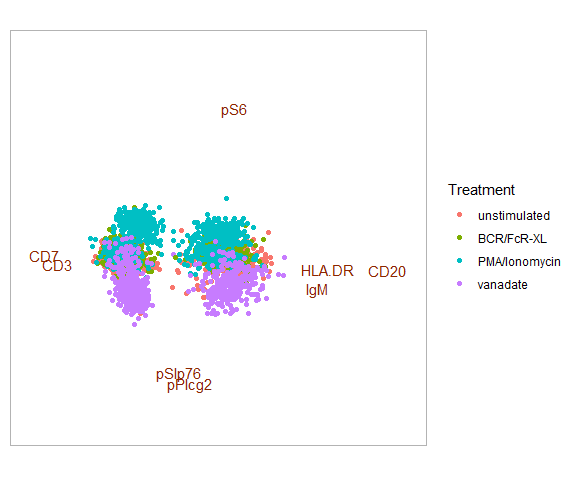# Building a functional T cells graph

## Building a cell-level distance matrix

We compute the cosine distance between cells based on all available channels:

btcells.dist <- as.matrix(btcells.df[,btcells.channels])
rownames(btcells.dist) <- btcells.df$cellID btcells.dist <- btcells.dist%*% t(btcells.dist) btcells.dist <- btcells.dist/(sqrt(diag(btcells.dist)) %*% t(sqrt(diag(btcells.dist)))) btcells.dist[btcells.dist>1] <- 1 btcells.dist[btcells.dist<0] <- 0 btcells.dist <- 2*acos(btcells.dist)/pi diag(btcells.dist) <- NA # avoid self loops ## Extracting an adjacency matrix Next we build an adjacency matrix, selecting the 63 nearest neighbors foreach cell: K <- floor(nrow(btcells.df)^0.5) btcells.adj <- apply(btcells.dist,1,rank,na.last = TRUE) btcells.adj[btcells.adj<=K] <- btcells.dist[btcells.adj<=K] btcells.adj[btcells.adj>K] <- 0 For 4000 vertices (cells) 250326 edges are recovered. We can compare the distribution of distances overall with that of selected nearest neighbors: bind_rows(data.frame(value=btcells.dist[sample(1000)], Type='Overall', stringsAsFactors = FALSE), data.frame(value=btcells.adj[btcells.adj!=0][sample(1000)], Type='Nearest Neighbors', stringsAsFactors = FALSE)) %>% mutate(Type=factor(Type), Type=relevel(Type,'Overall')) %>% filter(!is.na(value)) %>% ggplot(aes(x=value))+ geom_histogram(aes(fill=Type), position = 'identity', bins=50, alpha=0.5)+ theme_light(base_size=16)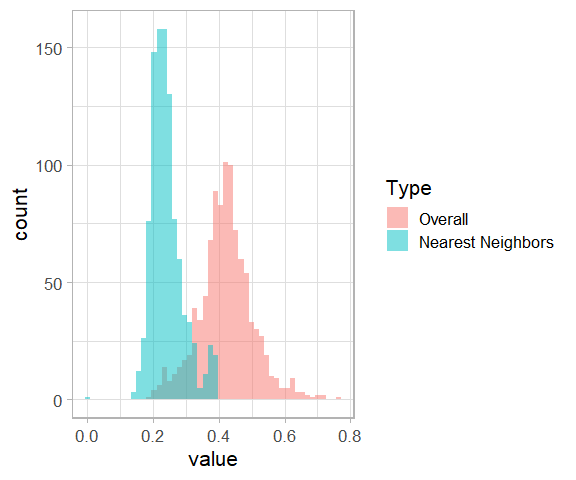We enriched for cells that are closer together than the average population. ## Computing edge weights from adjacency matrix Next we turn the distances into weights using a gaussian kernel: btcells.weights <- btcells.adj btcells.weights <- exp(-btcells.weights^2/(2*median(btcells.weights[btcells.weights!=0])^2)) btcells.weights[btcells.adj==0] <- 0 ## Building a kNN graph Next we build a kNN graph based on weights: btcells.graph <- graph_from_adjacency_matrix(btcells.weights, mode='undirected', weighted = TRUE, diag = FALSE) ## Extracting communities as functional states We use the Louvain algorithm to identify groups in the weighted T cells graph: btcells.groups <- cluster_louvain(btcells.graph) btcells.df <- btcells.df %>% ungroup() %>% mutate(Group=membership(btcells.groups), Group=as.numeric(Group), Group=factor(Group)) 6 communities were identified. # From communities to functional states btcells.df %>% gather('Channel','value', one_of(colnames(refPhenoMat),colnames(refFuncMat))) %>% filter(Channel %in% btcells.channels) %>% ggplot(aes(x=Channel,y=value))+ geom_fan()+ facet_grid(Group~.)+ theme_light(base_size = 16)+ theme(axis.text.x = element_text(angle = 45, hjust = 1)) ## Warning: guides(<scale> = FALSE) is deprecated. Please use guides(<scale> = ## "none") instead.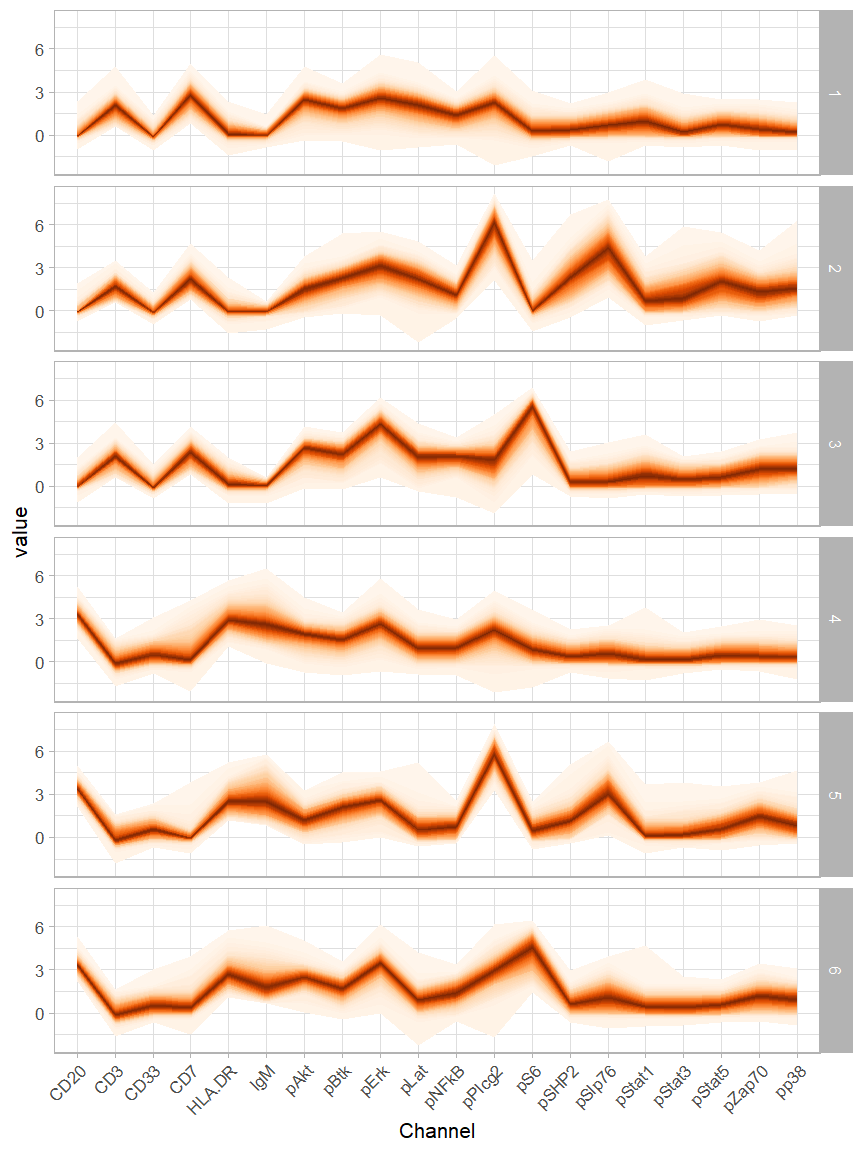Each community captures a specific population and a specific functional state, as illustrated in the following tables: btcells.df %>% count(Group,Cells) %>% group_by(Group) %>% mutate(n=n/sum(n)) %>% spread(Cells, n) ## # A tibble: 6 x 3 ## # Groups: Group  ## Group cd8+ igm+ ## <fct> <dbl> <dbl> ## 1 1 0.998 0.00166 ## 2 2 1 NA ## 3 3 1 NA ## 4 4 0.00114 0.999 ## 5 5 NA 1 ## 6 6 NA 1 And with respect to treatment: btcells.df %>% count(Group,Treatment) %>% group_by(Group) %>% mutate(n=n/sum(n)) %>% spread(Treatment, n) ## # A tibble: 6 x 5 ## # Groups: Group  ## Group unstimulated BCR/FcR-XL PMA/Ionomycin vanadate ## <fct> <dbl> <dbl> <dbl> <dbl> ## 1 1 0.392 0.401 0.139 0.0679 ## 2 2 0.0530 0.0243 0.00442 0.918 ## 3 3 0.00588 0.0147 0.976 0.00294 ## 4 4 0.536 0.131 0.191 0.142 ## 5 5 0.0406 0.00508 NA 0.954 ## 6 6 0.0166 0.528 0.455 NA # Using Graphviz to visualize functional graph Compared to Freeviz, where anchors are optimized based on classes, in Graphviz the anchors are optimized after the structure of the graph itself, similar to a force-directed layout with the context provided by anchors. As with Radviz and Freeviz we start with optimizing the anchors: btcells.S <- do.optimGraphviz(btcells.df[,btcells.channels],btcells.graph) ##  "# iters: 26" btcells.gv <- do.radviz(btcells.df, btcells.S, graph = btcells.graph) We can then plot the graph in the context of the selected channels, colored by community : plot(btcells.gv)+ geom_point(aes(color=Group))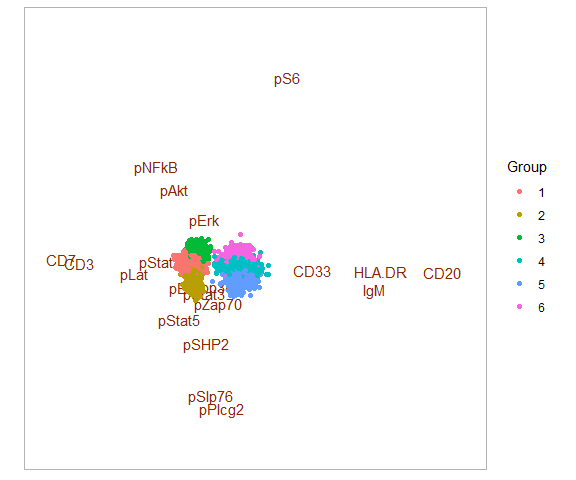This plot is to be compared to the classical force directed layout used to visualize weighted graphs: btcells.graph$layout <- layout_with_drl(btcells.graph)
community.cols <- hue_pal()(length(btcells.groups))
plot(btcells.graph,
vertex.label=NA,
vertex.color=community.cols[membership(btcells.groups)])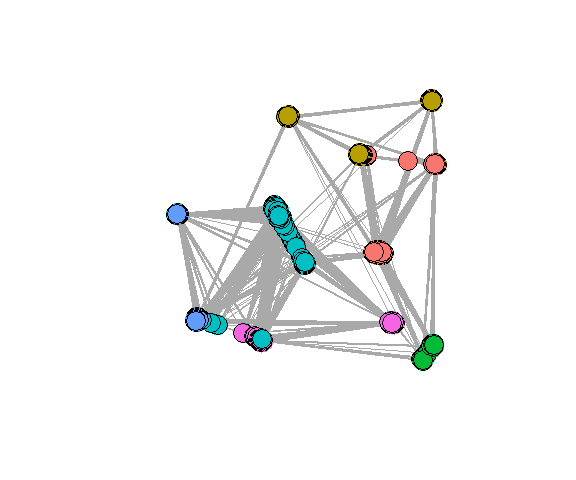Points can be colored by cell assignment:

plot(btcells.gv)+
geom_point(aes(color=Cells),alpha=0.5)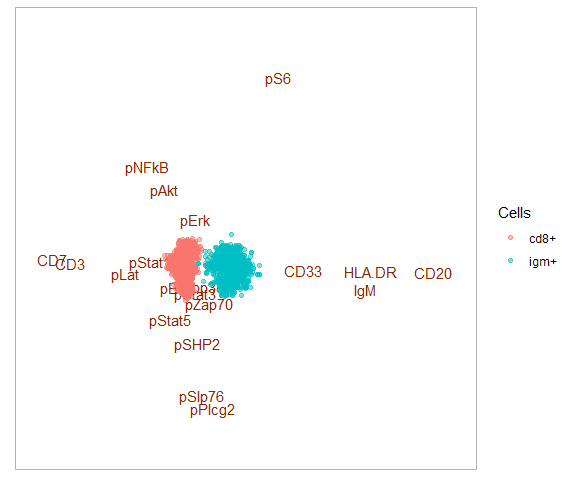Or treatment:

plot(btcells.gv)+
geom_point(aes(color=Treatment))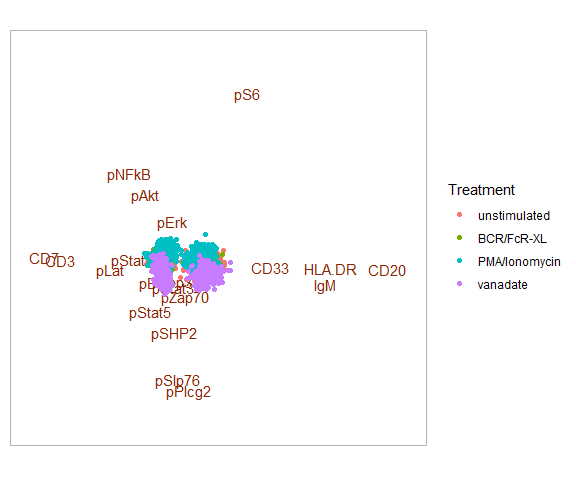Confirming previous observations made with fan plots. Compared to classical visualization, one can quickly identify channels associated with specific communities.